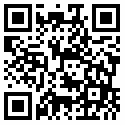## 350+ C Programming Examples

The C Examples based on real-time c examples. Which we are cover

1. Basic C Programming Examples.
2. Bitwise Operator Examples.
3. Conditional Operator Examples.
4. If Else Examples.
5. Switch Case Examples.
6. Loop & Iteration Examples.
7. Star Patterns Examples.
8. Number Pattern Exercises.
9. Function And Recursion Exercises.
10. Array And Matrix Ecercises.
11. String Exercises
12. Poiner Exercises
13. Files Handling Exercises

All the above examples are mainly focused on self-improve in C.

First, Basic Programming helps you to improve basic writing skill in an area of a circle, an area of a rectangle, perimeter of circle etc....

Second, In Bitwise Operator here you evaluate LSB, MSB, get an nth bit of a number, get the highest set bit of a number, convert one number system to another number system.

Third, In Conditional Operator Examples contains maximum between two numbers, three numbers, even and odd leap Year etc....

Forth, In If-Else Examples, check whether a number is positive, negative or zero, check whether a number is divisible by 5 and 11 or not, whether a number is even or odd, check vowel or Not, check Uppercase or Not, input week number and print weekday, input all sides of triangle etc....

Fifth, Switch Case Examples, Using Switch case print day of the week, print the total number of days in a month using a switch, maximum between two numbers and many more.

Sixth, Loop and Iteration Exercises contains different print statements from 1-100 even, odd, alphabets etc.

Seventh, Star Patterns contains different star patterns design like * to print in output.

Eighth, Number Patterns contains different number sequences in display time.

Ninth, Functions and Recursions Contain different function types and how it will help us to break large programs in smaller part using function and recursion.

Tenth, Array And Matrix Exercises contains different array operation and matrix operation.

Eleventh, String Exercises contains the different inbuilt string and without using string function.

Twelveth, Pointer Exercises initialize and use of pointers, add two numbers, swap two numbers, input and print array elements, reverse the array, add two matrices using a pointer.

Thirteenth, Files Handling Exercises create a file and write contents, save and close the files, read file contents and display on the console, read numbers from a file and write even, odd and prime numbers etc...
User Reviews
Based on 0 Votes and 0 User Reviews
5 Star
0
4 Star
0
3 Star
0
2 Star
0
1 Star
0